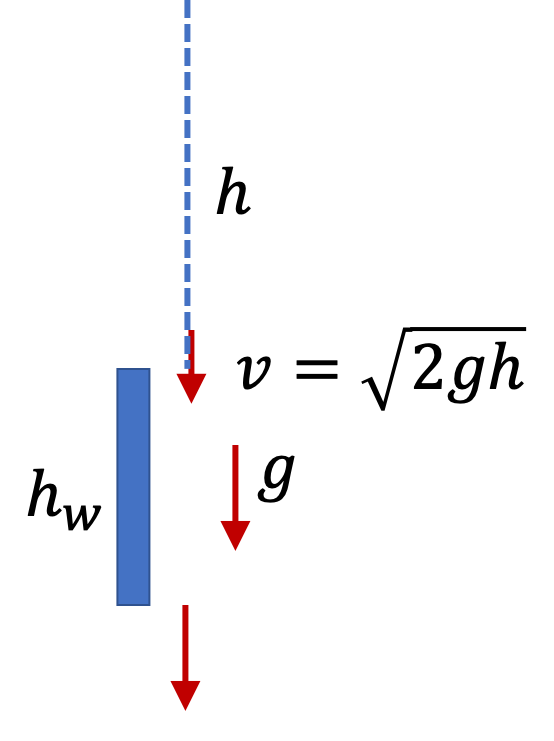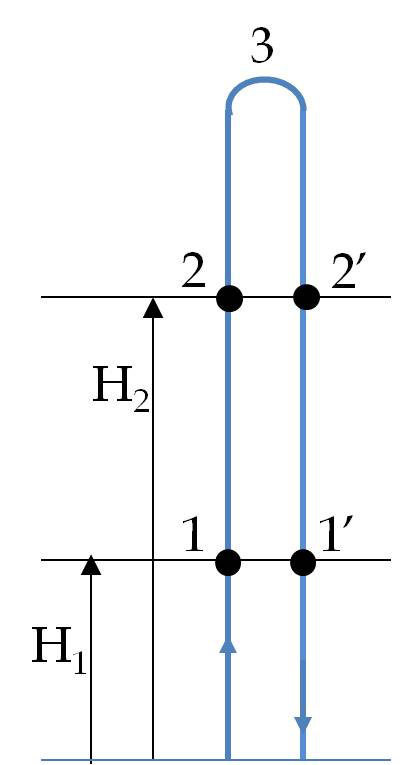## Section4.10Kinematics Bootcamp

### Subsection4.10.9Miscellaneous

Suppose you live on the $5^{\text{th}}$ floor of a tall building. Your friend goes to the roof and drops a steel ball from rest while you time the ball as it falls. You find that the ball takes $0.11\ \text{sec}$ to fall from the top of the window to the bottom of the window, a distance of $0.8$ meter. How high above the top of the window of the $5^{\text{th}}$ floor is the roof?

Hint

First find the speed at the top of the window. Then, use that as initial speed for the problem for just the window.

$2.31\text{ m}\text{.}$

Solution

Let $h$ be the height from top of the window to where the ball was let go from rest. We know that after freely falling this height, the ball would be moving at $\sqrt{2gh}\text{.}$

Now, let us look at motion from top of the window to bottom of the window. Let $t=0$ at the top of window and $t=t$ at tje bottom.Then, using positive $y$ axis pointed up, we have the following

\begin{equation*} v_{yi} = - \sqrt{2gh},\ \ a_y = -g,\ \ \Delta t= t, \ \ y_f-y_i = -h_w. \end{equation*}

Therefore we have the following equation of constant accleration useful in this context.

\begin{equation*} y_f - y_i = v_{yi}\Delta t + \dfrac{1}{2}a_y (\Delta t)^2. \end{equation*}

This gives

\begin{equation*} -h_w = -(\,\sqrt{2gh}\,)\, t -\dfrac{1}{2} g t^2. \end{equation*}

Solving for $\sqrt{2gh}$ we get

\begin{align*} \sqrt{2gh}\amp = \dfrac{h_w}{t} - \dfrac{1}{2} g t,\\ \amp = \dfrac{0.8}{0.11} - \dfrac{1}{2} \times 9.81\times 0.11 = 7.27 - 0.54 = 6.73. \end{align*}

Therefore,

\begin{equation*} h = \dfrac{6.73^2}{2\times 9.81} = 2.31\text{ m}. \end{equation*}

A football leaves the kicker's foot at $50^{\circ}$ from the horizontal direction. The football is required to clear a horizontal bar at a height of $10\text{ m}$ above the ground at a horizontal distance of $40\text{ m}\text{.}$ Find speed with which ball must leave the kicker's foot so that it would barely go over the bar.

Hint

Standard projectile motion setup.

$22.5\text{ m/s}\text{.}$

Solution

Let $h$ denote the height to clear and $D$ the horizontal distance to travel from the launch site as shown in Figure 4.10.49. Let $v_i$ denote the speed at the initial instant and $\theta$ angle of launch.

With $t$ the duration of the flight we have the following two equations, one along $x$ axis with $a_x=0$ and one along $y$ with $a_y=-g$ that is useful to solve this problem.

\begin{align} D \amp = v_i\, t\, \cos\,\theta,\label{eq-football-clearing-goal-x}\tag{4.10.1}\\ h \amp = v_i\, t\, \sin\,\theta -\dfrac{1}{2}g t^2,\label{eq-football-clearing-goal-y}\tag{4.10.2} \end{align}

replace $v_i t$ in the second equation to obtain an equation in $t$ that we can solve for $t\text{.}$

\begin{equation*} h = D \tan\,\theta - \dfrac{1}{2}g t^2, \end{equation*}

which gives

\begin{equation*} t^2 = \dfrac{2}{g}(D \tan\,\theta - h ) = (2/9.81)\times(40\tan\,50^\circ - 10 ) = 7.68. \end{equation*}

Therefore,

\begin{equation*} t = \sqrt{7.68} = 2.77\text{ s}. \end{equation*}

Using this in Eq. (4.10.1) we get speed at initial instant to be

\begin{equation*} v_i = \dfrac{D}{t \cos\,\theta} = \dfrac{40}{2.77\, \cos\,50^\circ} = 22.5\text{ m/s}. \end{equation*}

A rocket is rising at a constant velocity of $200\ \text{m/s}\text{.}$ A piece of the rocket comes loose and falls freely to the ground. It takes $40\ \text{sec}$ for the piece to fall to the ground after dislodging from the rocket. How far above the ground the piece came loose?

Hint

Use postive $y$ axis pointed up and use correct signs for directions.

$152\text{ m}\text{.}$

Solution

Let positive $y$ axis point up. Let $h$ be the required height. Then, we have the following informaion of the free motion during the interval $\Delta t=50\text {sec}\text{.}$

\begin{align*} \amp y_i = h, \ y_f=0,\ v_{iy} = +200\text{ m/s},\ v_{fy} =?,\\ \amp a_y=-9.81\text{ m/s}^2,\ \ t = 40\text{ s}. \end{align*}

Using the displacement-time equation we get

\begin{equation*} 0-h =200\times 40 - \dfrac{1}{2}\times 9.81 \times 40^2. \end{equation*}

Solving for $h$ we get

\begin{equation*} h = 152\text{ m}. \end{equation*}

One way to measure the value of $g$ is to launch a projectile vertically upward and record the return times at two different heights. Let $T_1$ and $T_2$ be the two return times, i.e.,the time for 1-3-1' and 2-3-2', at heights $H_1$ and $H_2$ respectively as shown in Fig. \ref{fig:prob-kin-8}. Deduce the following formula for $g$ from the heights and times.

\begin{equation*} g = \frac{8\left( H_2 - H_1\right)}{T_1^2 - T_2^2}. \end{equation*}Hint

With same launch find the initial speeds at the two heights. Then, use that to get hang times for each height.

Solution

Let $v_0$ be the launch speed, and $v_1$ and $v_2$ be speeds at heights $H_1$ and $H_2\text{.}$ Therefore we will have

\begin{align} v_1^2 \amp = v_0^2 - 2 g H_1 \label{eq-prob-kin-8-speed-height-1}\tag{4.10.3}\\ v_1^2 \amp = v_0^2 - 2 g H_1 \label{eq-prob-kin-8-speed-height-2}\tag{4.10.4} \end{align}

Now, when you launch a ball up with a speed $v$ and if the return time is $t\text{,}$ then you can set up the $y_f-y_i=0$ for this to show that.

\begin{equation*} 0 = v t - \dfrac{1}{2} g t^2. \end{equation*}

That is $gt$ of return time is

\begin{equation*} gt = 2 v. \end{equation*}

Applying this to the two return times we have

\begin{align} \amp gT_1 = 2 v_1,\tag{4.10.5}\\ \amp gT_2 = 2 v_2.\tag{4.10.6} \end{align}

Square these and subtract to get

\begin{equation*} g^2 \left( T_1^2 - T_2^2 \right) = 4\left( v_1^2 - v_2^2\right). \end{equation*}

Now using $v_1^2$ and $v_2^2$ from Eqs. (4.10.3) and (4.10.4), we will get

\begin{equation*} g^2 \left( T_1^2 - T_2^2 \right) = 8\, g\, \left(H_2 - H_1\right). \end{equation*}

Therefore,

\begin{equation*} g = \dfrac{ 8\, \left(H_2 - H_1\right) }{ T_1^2 - T_2^2 }. \end{equation*}